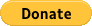## Exercise 1C Question 11

{(3^3)^2 × (4^2)^3 × (5^3)^2} / {(3^2)^3 × (4^3)^2 × (5^2)^3} is

## Donate in USD